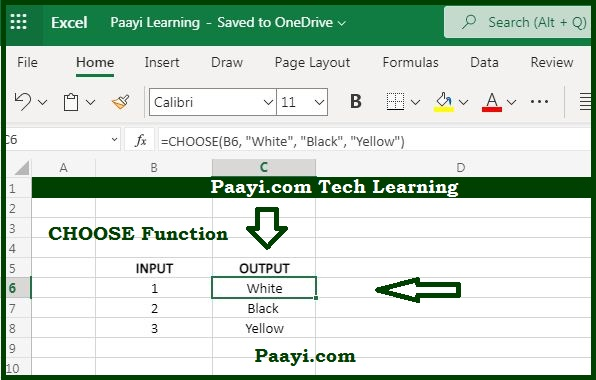# Learn How to Use Microsoft Excel CHOOSE Function

Written by | 0 Comments | 639 Views

In this article, you will learn how to use the Microsoft Excel CHOOSE function and its prime function in Microsoft Excel. You will also get to know the Microsoft Excel CHOOSE function return value and syntax with the help of some examples.

## Microsoft Excel CHOOSE Function

With the help of the Microsoft Excel CHOOSE function, you can get the value from a list based on a position. That means the CHOOSE function returns a value from a given list using a given position or index. Let's say, for example, the formula CHOOSE(2, "white", "black", "yellow") returns black, as blue is the second value listed after the index number. You can also include the references while providing value to the CHOOSE function.

### CHOOSE Function Return Value

It will return the value at the given position.

### CHOOSE Function Syntax

=CHOOSE (index-num, value1, [value2], ...)

Where the arguments:

• index-num: It is the value to choose, the number between 1 to 254.
• value1: The first value from where to select.
• value2: The second value from which you want to choose (optional).

## How to USE Microsoft Excel CHOOSE Function?As we know, the MS Excel CHOOSE function returns the value from a list provided using a given position or index. You can also provide the references while providing the value to the function. But you can't be able to retrieve the item from the inside range or array constant provided as a value using CHOOSE function. You can handle up to 254 values using CHOOSE function, but for managing more value sets, you can also use the INDEX function or INDEX with MATCH function.

Let us understand the function of CHOOSE, with the help of examples given below:

• CHOOSE(2, "white", "black", "yellow") - This will return black.
• CHOOSE(3, "white", "black", "yellow") - This will return yellow.